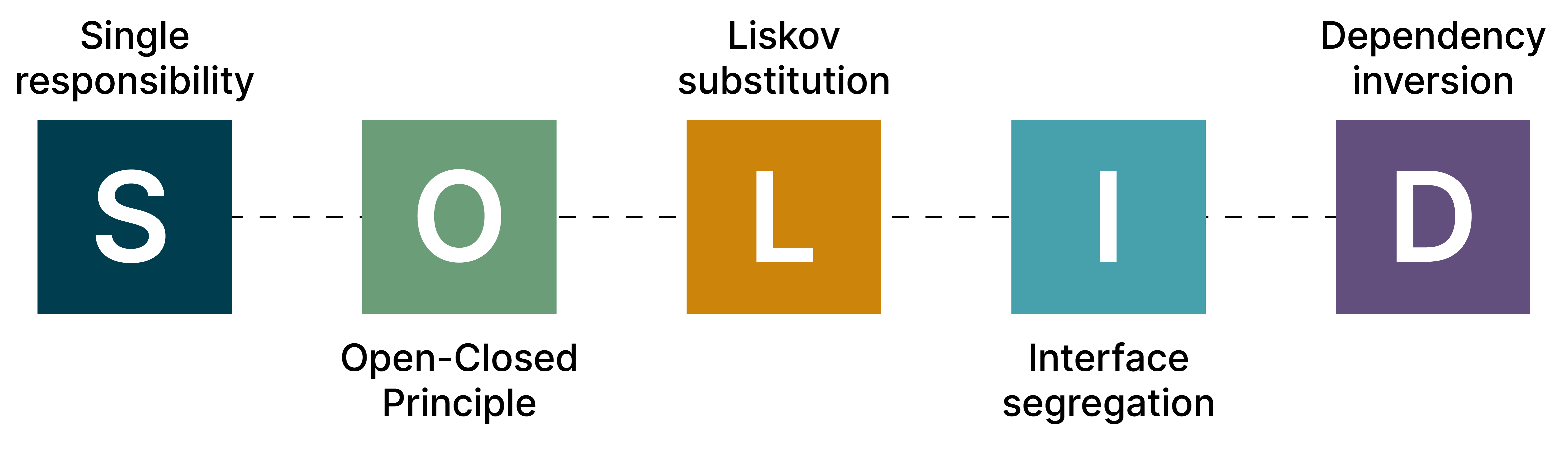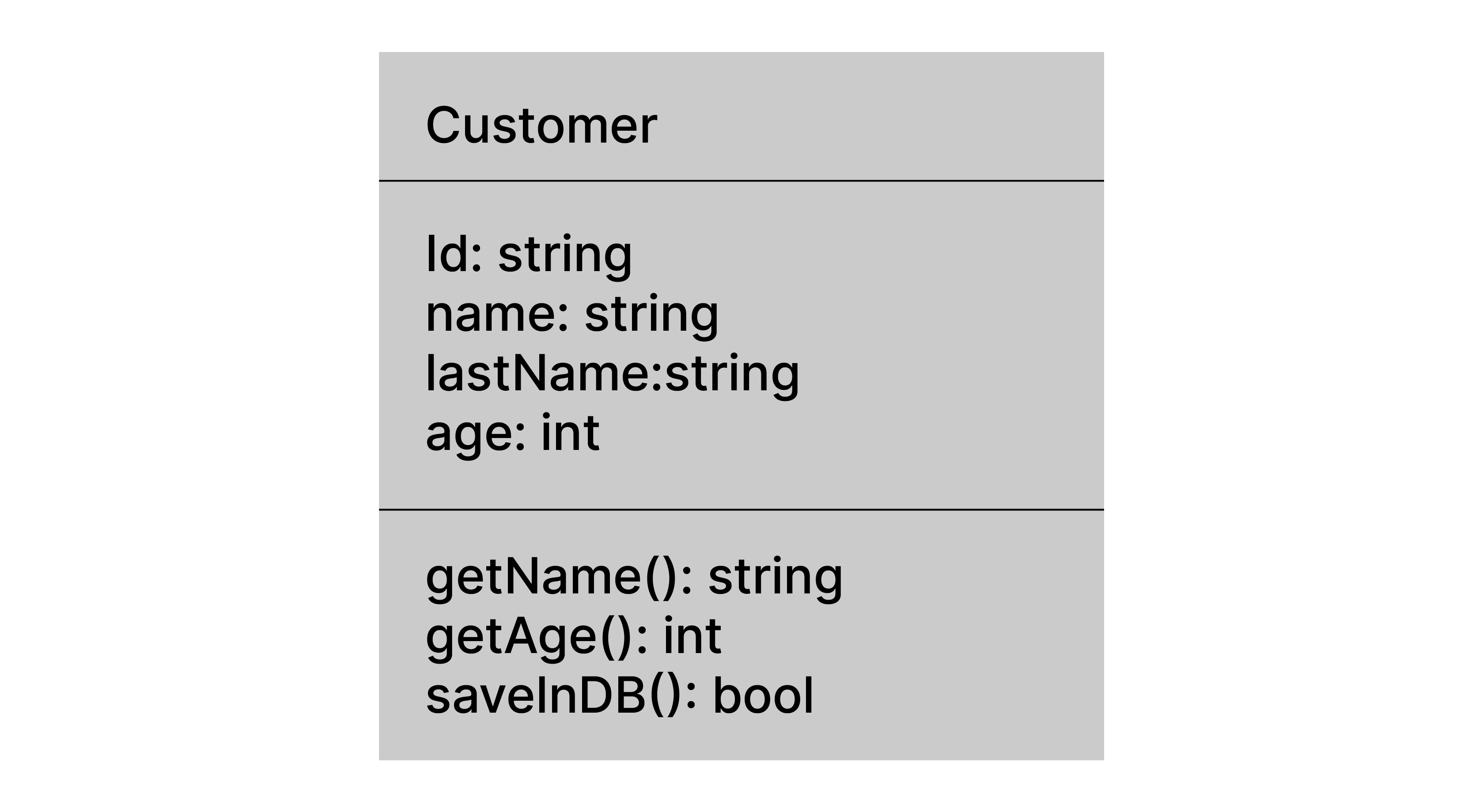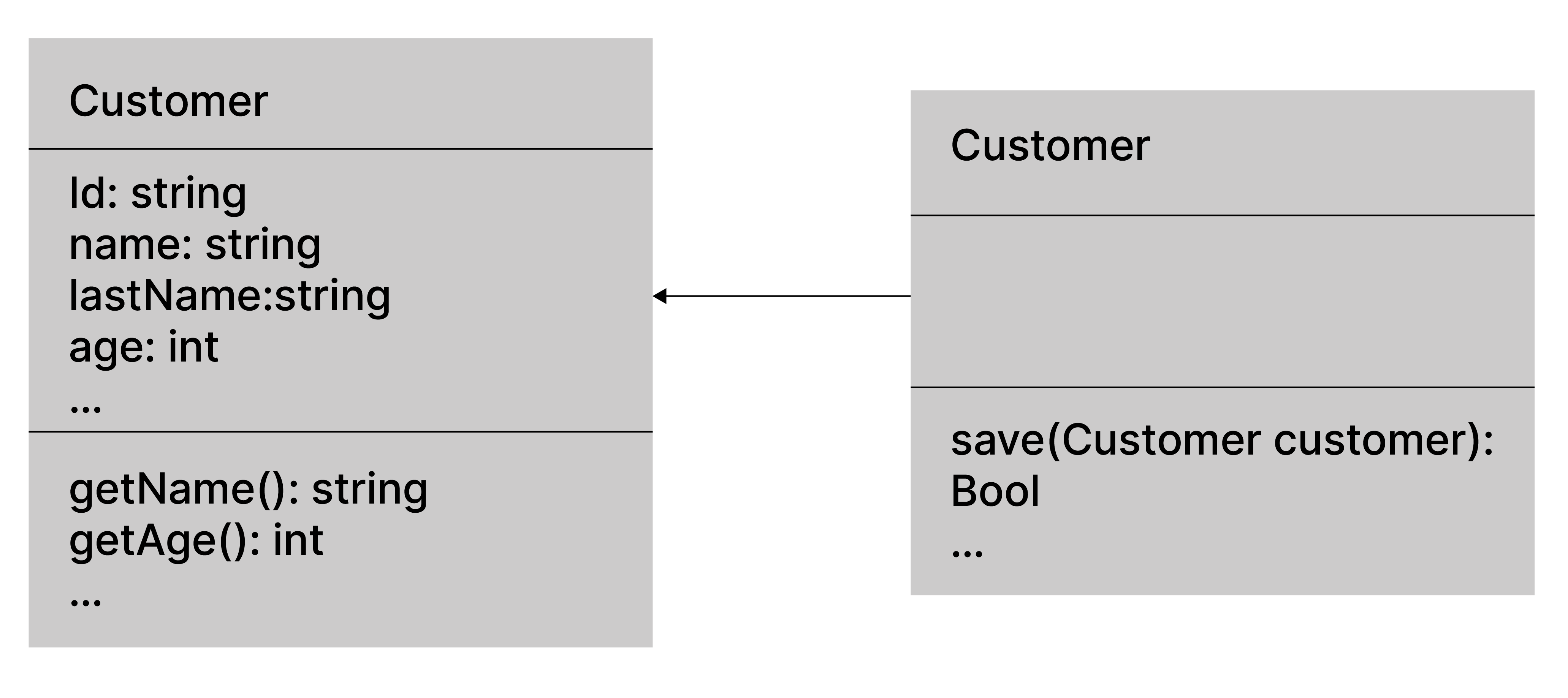Enable javascript in your browser for better experience. Need to know to enable it? Go here.
•Australia
•Brazil
•Canada
•Chile
•China
•Ecuador
•Germany
•India
•Singapore
•Spain
•Thailand
•United Kingdom
•United States
•Global

# SOLID Principles: How to create a code that is easy to extend and maintain

Part 1Object Oriented Programming, like other programming paradigms, was created initially to standardize the pillars, syntax and rules to be able to create a code in an understandable standard format and thus avoid falling into the creation of a spaghetti of variables, functions and classes flying between files and folders that in the end will become a difficult creature to tame, imagine then having to include a new functionality, it would be a challenge for the brave.

These problems are fought from an early stage of the project by including practices and structures that are tested, certified and adopted by developers in the world that ensure a clean structure, and that is easy to maintain and grow, which are called design patterns.

We will talk about a set of these patterns which are known as SOLID principles, a term coined by  Robert C. Martin applied initially for object-oriented programming (OOP) paradigm software development, which, when it’s being applied correctly, promotes the cohesion of the different elements of the software and, in turn, makes these elements as less coupled as possible.

SOLID, is the union of the initials of five design patterns which are the (S)ingle responsibility, (O) pen/ Close, (L) iskov Substitution, (I) nterface segregation and (D) ependency Injection principles.

## SRP: Single Responsibility Principle

The Single Responsibility Principle is the principle that promotes that your functions, classes or modules should have a single responsibility and that they should only change for a single reason.

This does not mean that a class or function can do only one thing, actually what this principle contemplates is that changes to an element of the code must be related to each other under the same concept, actor or context.

The following example reflects a case in which we have a customer (Customer) that, apart from encapsulating the attributes related to a customer, such as the identifier, first and last name, it can also perform operations on the database, breaking the principle of simple responsibility since this class can change either because the concept of client evolves (adding new attributes) as well as changing the way it is saved (changing from a database to Rest, among others).In this case, the responsibility of the database can be decoupled to another context in charge of persisting the information of a client, integrating new patterns such as the repository.Two symptoms that can be mentioned to identify the presence of a violation of the principle of single responsibility can be the accidental duplication that occurs when several actors interact with the same component for two different purposes. In the previous example we can denote as actors one in charge of building a Customer entity from the class definition and another that only requires saving it.

### Merge

This symptom is very common in collaborative projects, where several people work on the same code base with a version manager such as Git.

A situation can be presented, taking the previous example, that one person includes a new attribute to the client (telephone number) and another person changes the saving process of the client in the database, to a call to a web service.

When both people send their changes and at least one of them is mixed in the main branch, it will happen that the other person will have conflicts in their branch (merge conflict), if this happens but both occur with very different topics (one is to include an attribute , and the other communication with a web service), then we detect a conflict of the principle of simple responsibility.

A very important point of this principle is that it pushes to develop always thinking about the cohesion of what is developed, cohesion refers to the union of things that maintain a close relationship, that is, if I introduce a new change in the class Customer said change must be according to the concept of Customer, as a new attribute, or an action that is consistent for a customer.

## OCP: Open Close Principle

Formulated by Bertrand Meyer in 1988, the open / closed principle (OCP) states that the behaviors of an entity (class, module, function) must be open for extension but closed for modification. That is, write code that does not change when adding new features to the system.

Let's see an example of an application that calculates the area of ​​a geometric figure.

In the first version, it was requested that the application should be able to calculate the area of ​​a rectangle, and a Circle, so an example could be:

```class Rectangle {
```
``` int width, height;
```
```
```
``` Rectangle(int width, int height) {
```
```     this.width = width;
```
```     this.height = height;
```
``` }
```
```
```
``` public int getWidth() { return this.width;}
```
``` public int getHeight() { return this.height;}
```
```}
```
```
```
```class Circle {
```
``` float radius;
```
```
```
``` Circle(float radius) { this.radius = radius;}
```
```
```
``` public float getRadio() { return this.radius;}
```
```}
```
```
```
```
```
```public class Main {
```
```
```
``` public static void main(String[] args) {
```
```     Main program = new Main();
```
```     program.start ();
```
``` }
```
```
```
``` public void start() {
```
```     Circle circle = new Circle(5);
```
```     Rectangle rectangle = new Rectangle(5,10);
```
```     printArea (circle);
```
```     printArea (rectangle);
```
``` }
```
```
```
``` public static <T> void printArea(T geometricShape) {
```
```     if(geometricShape instanceof Circle) {
```
```         System.out.println (Math.PI * ((Circle) geometricShape) .getRadio());
```
```     } else {
```
```         Rectangle rectangle = (Rectangle) geometricShape;
```
```         System.out.println (rectangle.getWidth () * rectangle.getHeight());
```
```     }
```
``` }
```
```}
```

The Open/Closed principle is not fulfilled for this example, since the method printArea determines, based on which geometric figure is sent (Rectangle or Circle) which strategy to apply to calculate the area, this does not allow scaling the system , since that when you want to include new geometric figures (rectangle triangle for example.) with different ways of calculating their area, the if-else nest of the method printArea will grow indefinitely.

How can we solve this problem? As the Open/Closed principle says ("Open for extension, closed for modification"), we must find a way for the method to printArea abstract itself from the responsibility of deciding how to calculate the area, to this we can define an interface that represents the geometric figures as a whole, and that has the method calculateArea, each geometric figure implements this method to its shape and then printArea will only wait for an implementation of the interface GeometricFigure and execute the respective method without actually knowing how it is calculated. We will see this later with the Dependency Inversion Principle (DIP) in more detail.

Note: In Java, an interface is a class that abstracts a group of methods related to each other (by concept of domain, by pattern (eg a Client Repository), etc.) and defines the parameters that each method needs in addition to what they return defining a contract, but does not define the logic to follow to fulfill the contract (implementation).

```interface GeometricShape {
```
``` double calculateArea();
```
```}
```
```class Rectangle implements GeometricShape{
```
``` int width, height;
```
```

```
``` Rectangle(int width, int height) {
```
```     this.width = width;
```
```     this.height = height;
```
```

```
``` }
```
```

```
``` public int getWidth() { return this.width;}
```
``` public int getHeight() { return this.height;}
```
```

```
``` @Override
```
``` public double calculateArea() {
```
```     return this.width * this.height;
```
``` }
```
```}
```
```

```
```class Circle implements GeometricShape {
```
``` float radius;
```
```

```
``` Circle(float radius) { this.radius = radius;}
```
```

```
``` public float getRadius() { return this.radius;}
```
```

```
``` @Override
```
``` public double calculateArea() {
```
```     return Math.PI * this.radius;
```
``` }
```
```}
```
```

```
```class RectangleTriangle implements GeometricShape {
```
``` private int oh, ah;
```
```

```
``` public RectangleTriangle(int oh, int ah) {
```
```     this.oh = oh;
```
```     this.ah = ah;
```
``` }
```
```

```
``` @Override
```
``` public double calculateArea() {
```
```     return (this.oh *this.ah) /2;
```
``` }
```
```}
```
```

```
```

```
```public class Main {
```
```

```
``` public static void main(String[] args) {
```
```     Main program = new Main();
```
```     program.start ();
```
``` }
```
```

```
``` public void start() {
```
```     Circle circle = new Circle(5);
```
```     Rectangle rectangle = new Rectangle(5,10);
```
```     RectangleTriangle rectangleTriangle = new RectangleTriangle(5,2);
```
```     printArea (circle);
```
```     printArea (rectangle);
```
```     printArea (rectangleTriangle);
```
``` }
```
```

```
``` public static void printArea(GeometricShape shape) {
```
```         System.out.println (shape.calculateArea ());
```
``` }
```
```}
```

Here we eliminate the growth that the method of printing area was going to have, which increased for each new geometric figure that was created, to simply indicate that it was going to receive was a geometric Figure, and that the geometric figure defines having a method which is to calculate the area, this strategy of defining contracts in the form of interfaces and then declaring methods waiting for said contracts is a very common practice, it gives you the advantage of decoupling your main logic from implementations that could change, in a moment you could be printing the area of ​​a Circle, another moment a rectangle and thus, these types of practices open the door to using new design patterns such as the strategy pattern, where there are different strategies to address the same problem (the strategies are encapsulated under a context that are  rationale for the strategy in question).

In the second part of this article, we will delve into the LSP: Liskov Substitution Principle and the ISP: Interface Segregation Principle and how these principles are essential when creating a code that is easy to extend and maintain.

Disclaimer: The statements and opinions expressed in this article are those of the author(s) and do not necessarily reflect the positions of Thoughtworks.